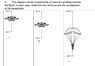# Determining net force and acceleration

Shaye
Homework Statement:
The diagram (attached) shows a parachutist of mass 82 kg falling towards
the Earth. In each case, determine the net force and the acceleration
of the parachutist
Relevant Equations:
F=Ma
Hi All,

Please see attached photo of the question.

It is asking for net force and acceleration. Taking the forces acting upwards and downwards on the
parachutist as vectors: for A the net force would be 800-300=500; B would be 0 and C; would that be 800-1500=-700? And if so would that imply that this is a negative acceleration of -8.5ms^-2 or is this a deceleration?

Thanks

#### Attachments

•Net Force and Acceleration.jpg
39.4 KB · Views: 244
•Delta2

Mentor
Homework Statement:: The diagram (attached) shows a parachutist of mass 82 kg falling towards
the Earth. In each case, determine the net force and the acceleration
of the parachutist
Relevant Equations:: F=Ma

Hi All,

Please see attached photo of the question.

It is asking for net force and acceleration. Taking the forces acting upwards and downwards on the
parachutist as vectors: for A the net force would be 800-300=500; B would be 0 and C; would that be 800-1500=-700? And if so would that imply that this is a negative acceleration of -8.5ms^-2 or is this a deceleration?

Thanks
Welcome to PhysicsForumsYou are on the right track (although I would include units with each of your equations and answers).

Think about what's going on in each of those 3 stages:
1. Initially the force of gravity is greater than air resistance, so the parachutist is speeding up in the fall
2. At terminal velocity, the forces of gravity and air resistance are equal
3. Right after pulling the ripcord, the force up is greater than the force of gravity down, so the parachutist decelerates
4. (Eventually the parachutist+parachute reach a new, slower terminal velocity)
Makes sense?

•Shaye
Homework Helper
Gold Member
2022 Award
Taking the forces acting upwards and downwards on the
parachutist as vectors
I think you mean "taking down as positive for forces and accelerations ".
would that imply that this is a negative acceleration of -8.5ms^-2 or is this a deceleration?
Since you have taken down as positive, this is an upward acceleration.
The term 'deceleration' is not much used in physics. In every day usage it means the speed is decreasing, i.e. the velocity and acceleration have opposite signs. So, yes, it is also a deceleration.

•Shaye
Shaye
Welcome to PhysicsForumsYou are on the right track (although I would include units with each of your equations and answers).

Think about what's going on in each of those 3 stages:
1. Initially the force of gravity is greater than air resistance, so the parachutist is speeding up in the fall
2. At terminal velocity, the forces of gravity and air resistance are equal
3. Right after pulling the ripcord, the force up is greater than the force of gravity down, so the parachutist decelerates
4. (Eventually the parachutist+parachute reach a new, slower terminal velocity)
Makes sense?

Thanks! My point of confusion has always been around the difference between deceleration and negative acceleration. Deceleration as you said is slowing down and negative acceleration just depends on what we take as positive or negative direction I guess

Shaye
I think you mean "taking down as positive for forces and accelerations ".

Since you have taken down as positive, this is an upward acceleration.
The term 'deceleration' is not much used in physics. In every day usage it means the speed is decreasing, i.e. the velocity and acceleration have opposite signs. So, yes, it is also a deceleration.
Cool! Thanks for this. Always needed to clarify in my head deceleration and negative acceleration

Homework Helper
Gold Member
... My point of confusion has always been around the difference between deceleration and negative acceleration.
The direction of the net force respect to the existing direction of movement is the key.
Negative or positive is just a convention of signs that you are free to establish.
http://hyperphysics.phy-astr.gsu.edu/hbase/Mechanics/motgraph.html#c2Last edited by a moderator:
•Shaye and berkeman
Shaye
The direction of the net force respect to the existing direction of movement is the key.
Negative or positive is just a convention of signs that you are free to establish.•